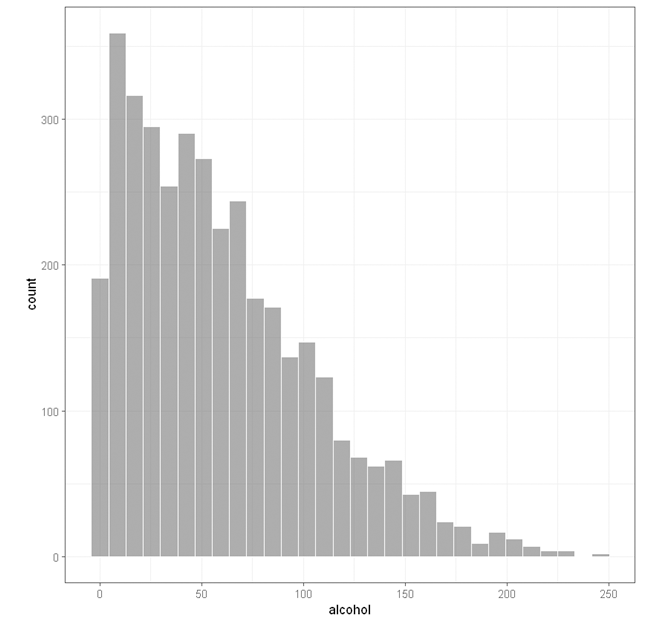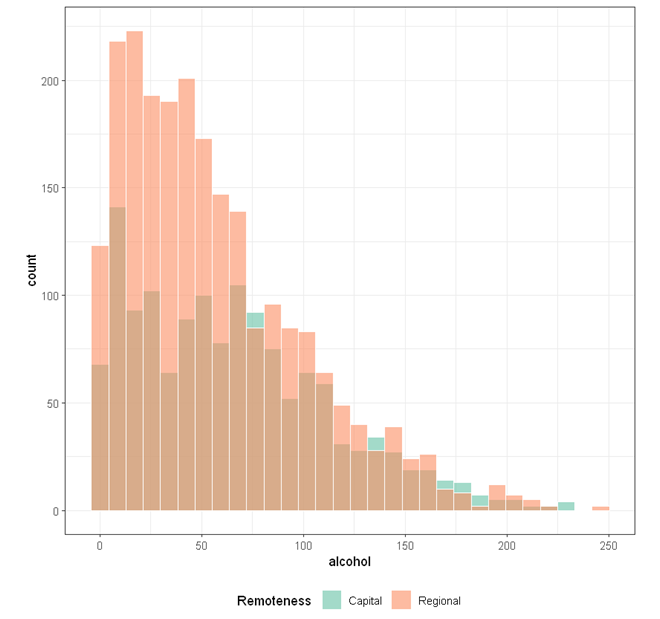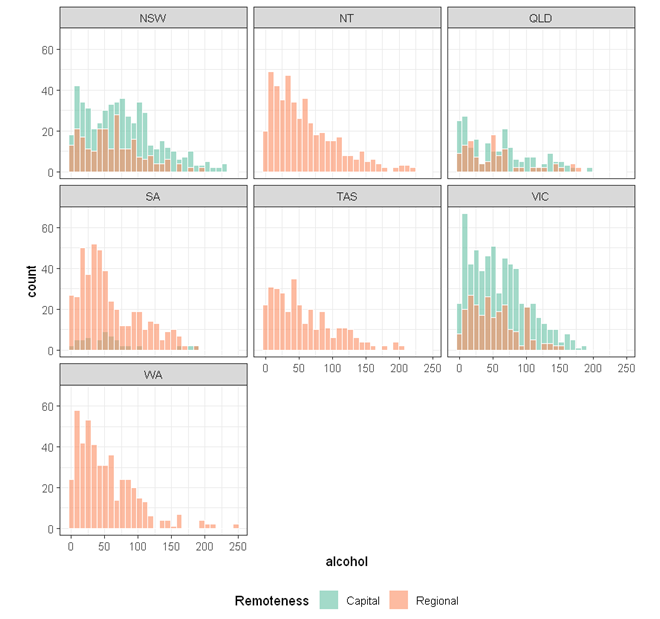# StatsNotebook

## Histogram

22 Oct, 2020 | 3 minutes read

Tags: DataViz, R code

The tutorial is based on R and StatsNotebook, a graphical interface for R.

Histogram can be used to show the distribution of numeric variable. StatsNotebook uses `geom_histogram()` from `ggplot2` to build density plot.

We use the built-in alcohol dataset in this example. This dataset can be loaded into StatsNotebook using instruction here. This is a simulated data of alcohol consumption from 3666 individuals.

This dataset can also be loaded using the following codes

``````library(tidyverse)
``````

We will use the following three variables from this dataset

1. alcohol - Number of standard drinks consumed in a month
2. Remoteness - Capital city or regional area
3. State - Seven states/territories in Australia: Queensland, New South Wales, Northern Territory, South Australia, Tasmania, Victoria and Western Australia.

In this example, we will build

To build a simple histogram for a single numeric variable (e.g. alcohol),

1. Click DataViz at the top
2. Click Numeric
3. Select Histogram from the menu
4. In the Histogram panel, select alcohol to Horizontal Axis.
5. Click Code and Run.
``````currentDataset %>%
ggplot(aes(x = alcohol)) +
geom_histogram(color = "white", alpha = 0.60, position = "identity", na.rm = TRUE)+
scale_fill_brewer(palette = "Set2")+
theme_bw(base_family = "sans")+
theme(legend.position = "bottom")

"Chan, G. and StatsNotebook Team (2020). StatsNotebook. (Version 0.1.0) [Computer Software]. Retrieved from https://www.statsnotebook.io"
"R Core Team (2020). The R Project for Statistical Computing. [Computer software]. Retrieved from https://r-project.org"
"Wickham H (2016). ggplot2: Elegant Graphics for Data Analysis. Springer-Verlag New York. ISBN 978-3-319-24277-4, https://ggplot2.tidyverse.org"
``````To build a histogram for a single numeric variable (e.g. alcohol) by groups (e.g. Remoteness),

1. Click DataViz at the top
2. Click Numeric
3. Select Histogram from the menu
4. In the Histogram panel, select alcohol to Horizontal axis and select Remoteness to Split by: Fill Color.
5. Click Code and Run.
``````currentDataset %>%
drop_na(Remoteness) %>%
ggplot(aes(x = alcohol, fill = Remoteness)) +
geom_histogram(color = "white", alpha = 0.60, position = "identity", na.rm = TRUE)+
scale_fill_brewer(palette = "Set2")+
theme_bw(base_family = "sans")+
theme(legend.position = "bottom")

"Chan, G. and StatsNotebook Team (2020). StatsNotebook. (Version 0.1.0) [Computer Software]. Retrieved from https://www.statsnotebook.io"
"R Core Team (2020). The R Project for Statistical Computing. [Computer software]. Retrieved from https://r-project.org"
"Wickham H (2016). ggplot2: Elegant Graphics for Data Analysis. Springer-Verlag New York. ISBN 978-3-319-24277-4, https://ggplot2.tidyverse.org"
``````To build histograms for a single numeric variable (e.g. alcohol) by groups (e.g. Remoteness) in multiple facets (by another variable, e.g. State),

1. Click DataViz at the top
2. Click Numeric
3. Select Histogram from the menu
4. In the histogram panel, select alcohol to Horizontal axis, select Remoteness to Split by: Fill Color and State to Facet.
5. Click Code and Run.
``````currentDataset %>%
drop_na(Remoteness, State) %>%
ggplot(aes(x = alcohol, fill = Remoteness)) +
geom_histogram(color = "white", alpha = 0.60, position = "identity", na.rm = TRUE)+
scale_fill_brewer(palette = "Set2")+
facet_wrap( ~ State)+
theme_bw(base_family = "sans")+
theme(legend.position = "bottom")

"Chan, G. and StatsNotebook Team (2020). StatsNotebook. (Version 0.1.0) [Computer Software]. Retrieved from https://www.statsnotebook.io"
"R Core Team (2020). The R Project for Statistical Computing. [Computer software]. Retrieved from https://r-project.org"
"Wickham H (2016). ggplot2: Elegant Graphics for Data Analysis. Springer-Verlag New York. ISBN 978-3-319-24277-4, https://ggplot2.tidyverse.org"
````````````Chan, G. and StatsNotebook Team (2020). StatsNotebook. [Computer Software]. Retrieved from https://www.statsnotebook.io
R Core Team (2020). The R Project for Statistical Computing. [Computer software]. Retrieved from https://r-project.org
Wickham H (2016). ggplot2: Elegant Graphics for Data Analysis. Springer-Verlag New York. ISBN 978-3-319-24277-4, https://ggplot2.tidyverse.org
``````